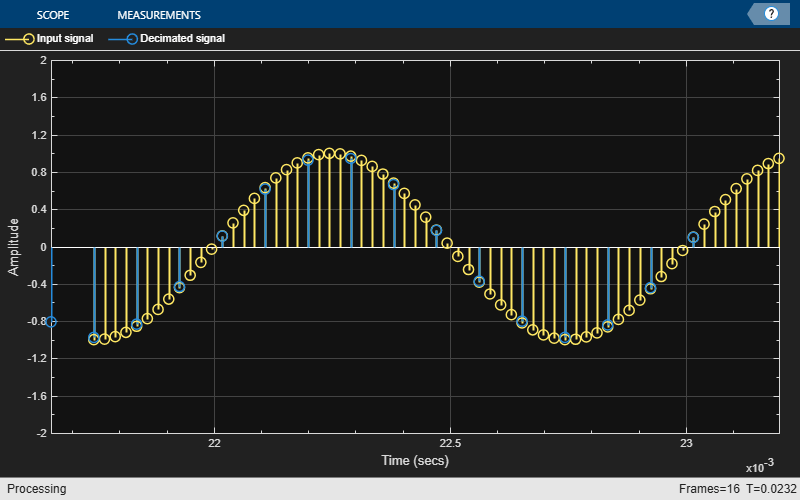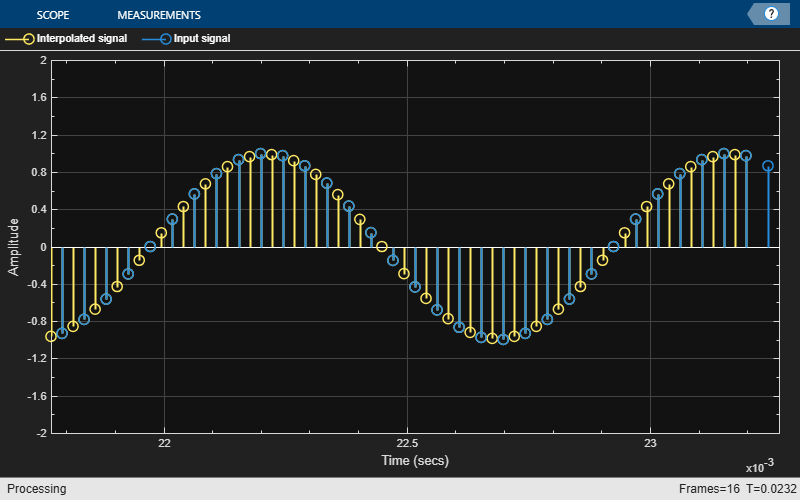# info

## Syntax

``s = info(sysobj)``
``s = info(sysobj,infoType)``
``s = info(___,'Arithmetic',arithType)``

## Description

example

````s = info(sysobj)` returns very basic information about the filter System object™. The particulars depend on the filter type and structure.```

example

````s = info(sysobj,infoType)` returns the amount of filter information as specified by the `infoType`.```
````s = info(___,'Arithmetic',arithType)` analyzes the filter System object, based on the arithmetic specified in `arithType`, using either of the previous syntaxes.For more input options, see `info` in Signal Processing Toolbox™.```

## Examples

collapse all

Obtain short-format and long-format information about a filter.

```d = fdesign.lowpass; f = design(d,'SystemObject',true); info(f)```
```ans = 6x35 char array 'Discrete-Time FIR Filter (real) ' '------------------------------- ' 'Filter Structure : Direct-Form FIR' 'Filter Length : 43 ' 'Stable : Yes ' 'Linear Phase : Yes (Type 1) ' ```
`info(f,'long')`
```ans = 45x45 char array 'Discrete-Time FIR Filter (real) ' '------------------------------- ' 'Filter Structure : Direct-Form FIR ' 'Filter Length : 43 ' 'Stable : Yes ' 'Linear Phase : Yes (Type 1) ' ' ' 'Design Method Information ' 'Design Algorithm : equiripple ' ' ' 'Design Options ' 'Density Factor : 16 ' 'Maximum Phase : false ' 'Minimum Order : any ' 'Minimum Phase : false ' 'Stopband Decay : 0 ' 'Stopband Shape : flat ' 'SystemObject : true ' 'Uniform Grid : true ' ' ' 'Design Specifications ' 'Sample Rate : N/A (normalized frequency) ' 'Response : Lowpass ' 'Specification : Fp,Fst,Ap,Ast ' 'Passband Edge : 0.45 ' 'Passband Ripple : 1 dB ' 'Stopband Edge : 0.55 ' 'Stopband Atten. : 60 dB ' ' ' 'Measurements ' 'Sample Rate : N/A (normalized frequency)' 'Passband Edge : 0.45 ' '3-dB Point : 0.46957 ' '6-dB Point : 0.48314 ' 'Stopband Edge : 0.55 ' 'Passband Ripple : 0.89042 dB ' 'Stopband Atten. : 60.945 dB ' 'Transition Width : 0.1 ' ' ' 'Implementation Cost ' 'Number of Multipliers : 43 ' 'Number of Adders : 42 ' 'Number of States : 42 ' 'Multiplications per Input Sample : 43 ' 'Additions per Input Sample : 42 ' ```

Note: If you are using R2016a or an earlier release, replace each call to the object with the equivalent step syntax. For example, `obj(x)` becomes `step(obj,x)`.

Create a `dsp.CICDecimator` System object™ with `DecimationFactor` set to 4. Decimate a signal from 44.1 kHz to 11.025 kHz.

```cicdec = dsp.CICDecimator(4); cicdec.FixedPointDataType = 'Minimum section word lengths'; cicdec.OutputWordLength = 16;```

Create a fixed-point sinusoidal input signal of 1024 samples, with a sampling frequency of 44.1e3 Hz.

```Fs = 44.1e3; n = (0:1023)'; % 0.0232 sec signal x = fi(sin(2*pi*1e3/Fs*n),true,16,15);```

Create a `dsp.SignalSource` object.

`src = dsp.SignalSource(x,64);`

Decimate the output with 16 samples per frame.

```y = zeros(16,16); for ii = 1:16 y(ii,:) = cicdec(src()); end```

Plot the first frame of the original and decimated signals. Output latency is 2 samples.

```gainCIC = ... (cicdec.DecimationFactor*cicdec.DifferentialDelay)^cicdec.NumSections; stem(n(1:56)/Fs,double(x(4:59))) hold on; stem(n(1:14)/(Fs/cicdec.DecimationFactor),double(y(1,3:end))/gainCIC,'r','filled') xlabel('Time (sec)') ylabel('Signal Amplitude') legend('Original signal','Decimated signal','Location','north') hold off;```Using the `info` method in `'long'` format, obtain the word lengths and fraction lengths of the fixed-point filter sections and the filter output.

`info(cicdec,'long')`
```ans = 'Discrete-Time FIR Multirate Filter (real) ----------------------------------------- Filter Structure : Cascaded Integrator-Comb Decimator Decimation Factor : 4 Differential Delay : 1 Number of Sections : 2 Stable : Yes Linear Phase : Yes (Type 1) Implementation Cost Number of Multipliers : 0 Number of Adders : 4 Number of States : 4 Multiplications per Input Sample : 0 Additions per Input Sample : 2.5 Fixed-Point Info Section word lengths : 20 19 19 18 Section fraction lengths : 15 14 14 13 Output word length : 16 Output fraction length : 11 ' ```

Note: If you are using R2016a or an earlier release, replace each call to the object with the equivalent `step` syntax. For example, `obj(x)` becomes `step(obj,x)`.

Create a `dsp.CICInterpolator` System object™ with `InterpolationFactor` set to 2. Interpolate a fixed-point signal by a factor of 2 from 22.05 kHz to 44.1 kHz.

`cicint = dsp.CICInterpolator(2)`
```cicint = dsp.CICInterpolator with properties: InterpolationFactor: 2 DifferentialDelay: 1 NumSections: 2 FixedPointDataType: 'Full precision' ```

Create a `dsp.SineWave` object with `SampleRate` set to 22.05 kHz, `SamplesPerFrame` set to 32, and `OutputDataType` set to `'Custom'`. To generate a fixed-point signal, set the `CustomOutputDataType` property to a `numerictype` object. For the purpose of this example, set the value to `numerictype([],16)`. The fraction length is computed based on the values of the generated sinusoidal signal to give the best possible precision.

To generate a fixed-point signal, set the `Method` property of the `dsp.SineWave` object to `'Table lookup'`. This method of generating the sinusoidal signal requires that the period of every sinusoid in the output be evenly divisible by the sample period. That is, $1/{\mathit{f}}_{\mathit{i}}{\mathit{T}}_{\mathit{s}}={\mathit{k}}_{\mathit{i}}$ must be an integer value for every channel i = 1, 2, ..., N. The value of ${\mathit{T}}_{\mathit{s}}$ equals $1/{\mathit{F}}_{\mathit{s}}$, the variable ${\mathit{f}}_{\mathit{i}}$ is the frequency of the sinusoidal signal, and ${\mathit{F}}_{\mathit{s}}$ is the sample rate of the signal. In other words, the ratio ${\mathit{F}}_{\mathit{s}}/{\mathit{f}}_{\mathit{i}}$ must be an integer. For more details, see the Algorithms section on the `dsp.SineWave` object page.

In this example, ${\mathit{F}}_{\mathit{s}}$ is set to 22050 Hz and ${\mathit{f}}_{\mathit{i}}$ is set to 1050 Hz.

```Fs = 22.05e3; sine = dsp.SineWave('Frequency',1050,'SampleRate',Fs,'SamplesPerFrame',32,... 'Method','Table lookup','OutputDataType','Custom')```
```sine = dsp.SineWave with properties: Amplitude: 1 Frequency: 1050 PhaseOffset: 0 ComplexOutput: false Method: 'Table lookup' TableOptimization: 'Speed' SampleRate: 22050 SamplesPerFrame: 32 OutputDataType: 'Custom' Show all properties ```

In each loop of the iteration, stream in a frame of the fixed-point sinusoidal signal sampled at 22.05 kHz. Interpolate the streamed signal by a factor of 2. The interpolated output has 64 samples per frame.

```for i = 1:16 x = sine(); y = cicint(x); end```

The output of the CIC interpolation filter is amplified by a specific gain value. You can determine this value using the `gain` function. This gain equals the gain of the $2{\mathit{N}}^{\mathrm{th}}$ stage of the CIC interpolation filter and equals ${\left(\mathit{I}×\mathit{D}\right)}^{\mathit{N}}/\mathit{I}$, where $\mathit{I}$ is the interpolation factor, $\mathit{D}$ is the differential delay, and $\mathit{N}$ is the number of sections of the CIC interpolator.

`gainCIC = gain(cicint)`
```gainCIC = 2 ```

To adjust this amplified output and to match it to the amplitude of the original signal, divide the CIC interpolated signal with the computed gain value.

Compare the last frames of the original and the interpolated signals. While plotting, account for the output latency of 2 samples.

```n = (0:63)'; stem(n(1:31)/Fs, double(x(1:31)),'r','filled') hold on; I = cicint.InterpolationFactor; stem(n(1:61)/(Fs*I), ... double(y(4:end))/gainCIC,'b') xlabel('Time (sec)') ylabel('Signal Amplitude') legend('Original Signal','Interpolated Signal',... 'location','north') hold off;```Using the `info` function in the `'long'` format, obtain the word lengths and fraction lengths of the fixed-point filter sections and the filter output.

`info(cicint,'long')`
```ans = 'Discrete-Time FIR Multirate Filter (real) ----------------------------------------- Filter Structure : Cascaded Integrator-Comb Interpolator Interpolation Factor : 2 Differential Delay : 1 Number of Sections : 2 Stable : Yes Linear Phase : Yes (Type 1) Implementation Cost Number of Multipliers : 0 Number of Adders : 4 Number of States : 4 Multiplications per Input Sample : 0 Additions per Input Sample : 6 Fixed-Point Info Section word lengths : 17 17 17 17 Section fraction lengths : 14 14 14 14 Output word length : 17 Output fraction length : 14 ' ```

## Input Arguments

collapse all

Amount of filter information to be displayed. When this property is set to:

• `'short'` –– The function displays the same information as `info(sysobj)`, which is the basic filter information.

• `'long'` –– The function returns the following information about the filter:

• Specifications such as the filter structure and filter order.

• Information about the design method and options.

• Performance measurements for the filter response, such as the passband cutoff or stopband attenuation, included in the `measure` method

.

• Cost of implementing the filter in terms of operations required to apply the filter to data, included in the `cost` method.

When the filter uses fixed-point arithmetic, the function returns additional information about the filter, including the arithmetic setting and details about the filter internals.

Data Types: `char` | `string`

Arithmetic used in the filter analysis, specified as `'double'`, `'single'`, or `'Fixed'`. When the arithmetic input is not specified and the filter System object is unlocked, the analysis tool assumes a double-precision filter. When the arithmetic input is not specified and the System object is locked, the function performs the analysis based on the data type of the locked input.

The `'Fixed'` value applies to filter System objects with fixed-point properties only.

When the `'Arithmetic'` input argument is specified as `'Fixed'` and the filter object has the data type of the coefficients set to `'Same word length as input'`, the arithmetic analysis depends on whether the System object is unlocked or locked.

• unlocked –– The analysis object function cannot determine the coefficients data type. The function assumes that the coefficients data type is signed, has a 16-bit word length, and is auto scaled. The function performs fixed-point analysis based on this assumption.

• locked –– When the input data type is `'double'` or `'single'`, the analysis object function cannot determine the coefficients data type. The function assumes that the data type of the coefficients is signed, has a 16-bit word length, and is auto scaled. The function performs fixed-point analysis based on this assumption.

To check if the System object is locked or unlocked, use the `isLocked` function.

When the arithmetic input is specified as `'Fixed'` and the filter object has the data type of the coefficients set to a custom numeric type, the object function performs fixed-point analysis based on the custom numeric data type.

## Output Arguments

collapse all

Filter information, returned as a character array.

When the `infoType` is `'short'`, the function displays basic filter information. When the `infoType` is `'long'`, the function displays the following information:

• Specifications such as the filter structure and filter order

• Information about the design method and options

• Performance measurements for the filter response, such as the passband cutoff or stopband attenuation, included in the `measure` method

• Cost of implementing the filter in terms of operations required to apply the filter to data, included in the `cost` method

When the filter uses fixed-point arithmetic, the function returns additional information about the filter, including the arithmetic setting and details about the filter internals.

### Topics

Introduced in R2011a

Watch now